## Example Questions

← Previous 1 3 4

### Example Question #445 : Algebra

Simplify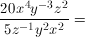None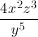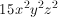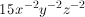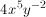Explanation:

Divide the coefficients and subtract the exponents.

### Example Question #2 : How To Divide Exponents

Which of the following is equal to the expression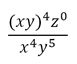, where

xyz ≠ 0?

1/y

z/(xy)

xyz

z

xy

1/y

Explanation:

(xy)4 can be rewritten as x4y4 and z0 = 1 because a number to the zero power equals 1.  After simplifying, you get 1/y.

### Example Question #447 : Algebra

If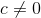, then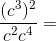Cannot be determined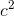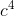Explanation:

Start by simplifying the numerator and denominator separately. In the numerator, (c3)2 is equal to c6. In the denominator, c2 * c4 equals c6 as well. Dividing the numerator by the denominator, c6/c6, gives an answer of 1, because the numerator and the denominator are the equivalent.

### Example Question #448 : Algebra

If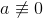, which of the following is equal to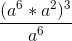?

a

a18

The answer cannot be determined from the above information

a4

a6

a18

Explanation:

The numerator is simplified to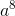(by adding the exponents), then cube the result. a24/a6 can then be simplified to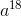.

### Example Question #1 : How To Divide Exponents

[641/2 + (–8)1/3] * [43/16 – 3171/3169] =

–5

9

30

16

–30

–30

Explanation:

Let's look at the two parts of the multiplication separately. Remember that (–8)1/3 will be negative. Then 641/2 + (–8)1/3 = 8 – 2 = 6.

For the second part, we can cancel some exponents to make this much easier. 43/16 = 43/42 = 4. Similarly, 3171/3169 = 3171–169 = 32 = 9. So 43/16 – 3171/3169 = 4 – 9 = –5.

Together, [641/2 + (–8)1/3] * [43/16 – 3171/3169] = 6 * (–5) = –30.

### Example Question #1 : Exponential Operations

Evaluate: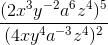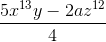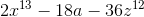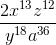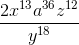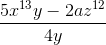Explanation:

Distribute the outside exponents first: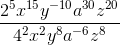Divide the coefficient by subtracting the denominator exponents from the corresponding numerator exponents:### Example Question #1 : Exponential Operations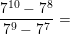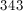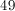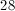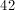Explanation:

The easiest way to solve this is to simplify the fraction as much as possible. We can do this by factoring out the greatest common factor of the numerator and the denominator. In this case, the GCF is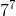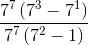Now, we can cancel out thefrom the numerator and denominator and continue simplifying the expression.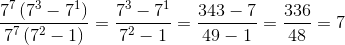### Example Question #81 : Exponents

Simplify:  y3x4(yx3 + y2x2 + y15 + x22)

y3x12 + y6x8 + y45 + x88

2x4y4 + 7y15 + 7x22

y3x12 + y12x8 + y24x4 + y3x23

y4x7 + y5x6 + y18x4 + y3x26

y3x12 + y6x8 + y45x4 + y3x88

y4x7 + y5x6 + y18x4 + y3x26

Explanation:

When you multiply exponents, you add the common bases:

y4 x7 + y5x6 + y18x4 + y3x26

### Example Question #3 : Exponential Operations

Indicate whether Quantity A or Quantity B is greater, or if they are equal, or if there is not enough information given to determine the relationship.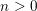Quantity A: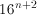Quantity B: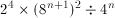The quantities are equal.

Quantity A is greater.

Quantity B is greater.

The relationship cannot be determined from the information given.

Quantity B is greater.

Explanation:

By using exponent rules, we can simplify Quantity B.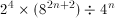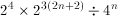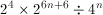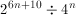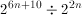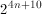Also, we can simplify Quantity A.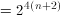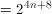Since n is positive,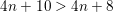### Example Question #4 : Exponential Operations

If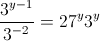, what is the value of?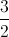Explanation:

Rewrite the term on the left as a product. Remember that negative exponents shift their position in a fraction (denominator to numerator).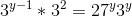The term on the right can be rewritten, as 27 is equal to 3 to the third power.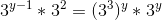Exponent rules dictate that multiplying terms allows us to add their exponents, while one term raised to another allows us to multiply exponents.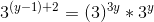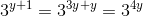We now know that the exponents must be equal, and can solve for.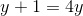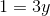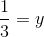← Previous 1 3 4

Tired of practice problems?

Try live online GRE prep today.